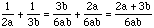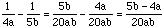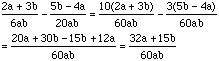Date: Wed, 30 Sep 1998 18:37:12 -0600 (CST) To: QandQ@MathCentral.uregina.ca Subject: Intergers and algerbra Name: Pam Who is asking: Student Level: Middle Question: Can you help me simplfy this? (1/2a + 1/3b) - (1/4a - 1/5b) + (1/6a - 1/7) thanx Hi Pam, To add fractions, wheteher they involve only integers or integers and letters, you need to first find a common denominator. For the first two fractions the denominators are 2a and 3b so for a common denominator you can use (2a)(3b) = 6ab. ThusFor the second pair of fractions the common denominator can be (4a)(5b) = 20ab, thusYou now have fractions with denominators 6ab and 20ab so 60ab is a common denominator, and henceNow you need to add the third pair and then add what you get to the sum you have already. Cheers Harley

Go to Math Central